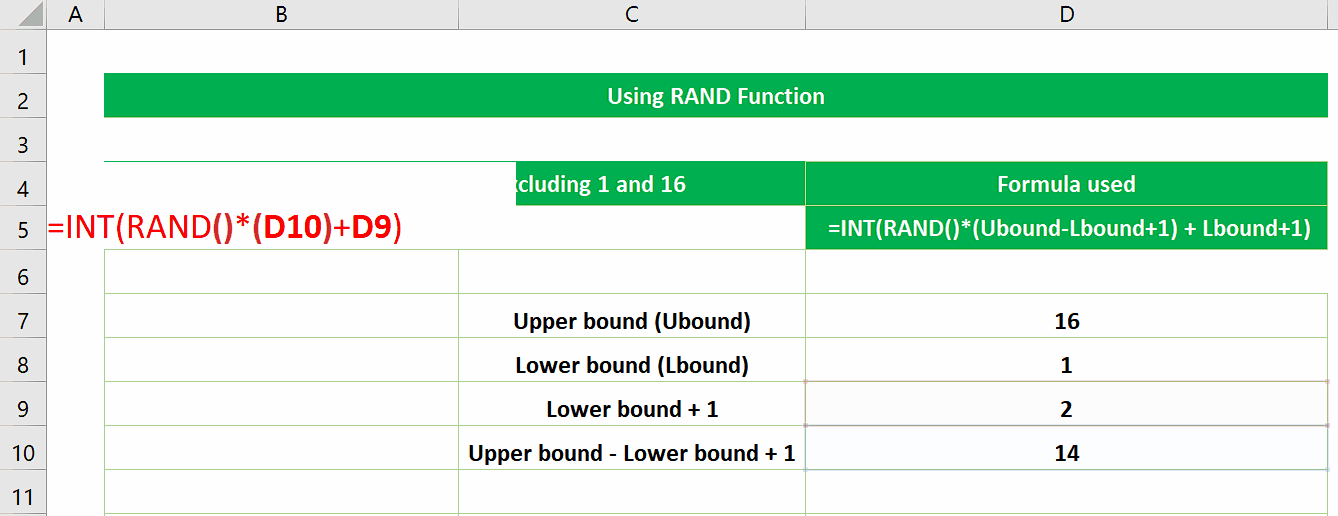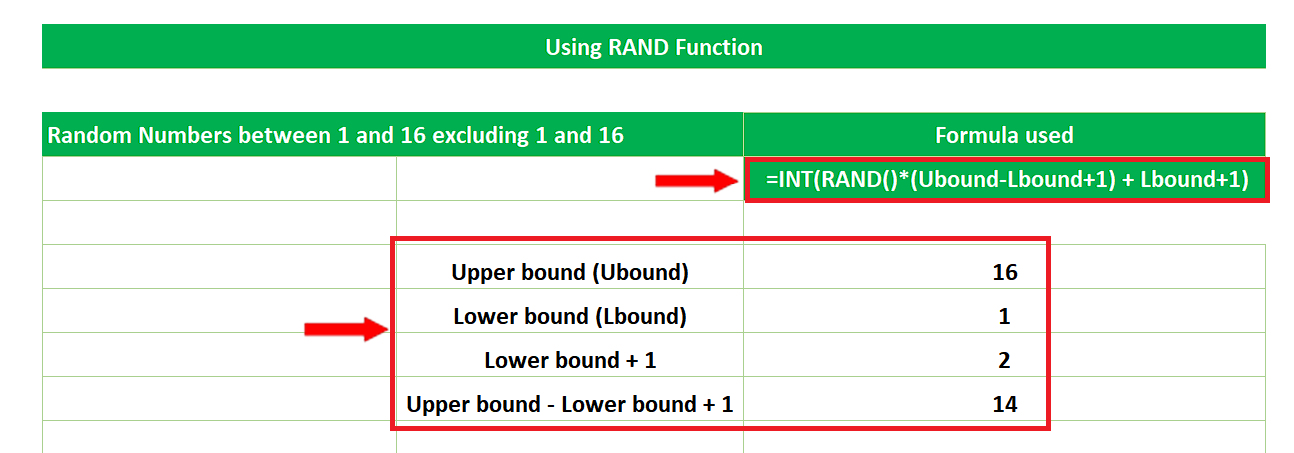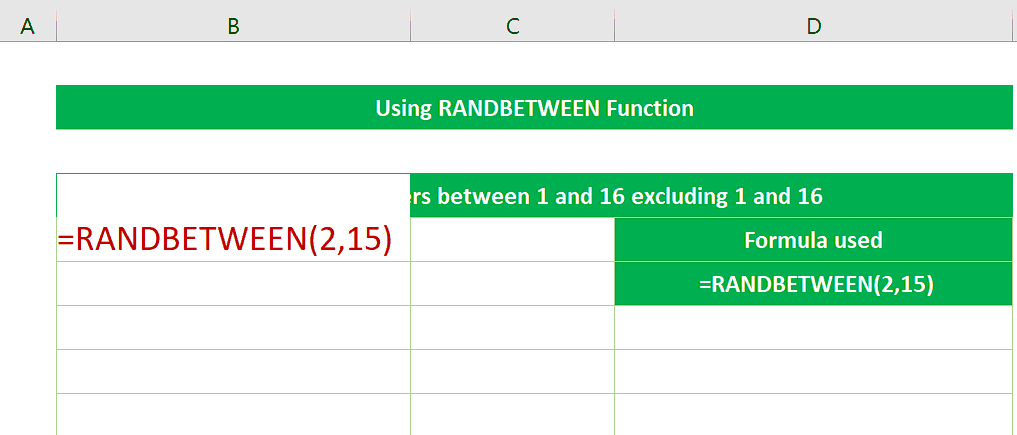# How to generate a random number between 1 to 16 in excel

In today’s tutorial we’ll learn how to generate random numbers between 1 to 16 which means that 1 and 16 will not be included in the list of random numbers. We’ll use the following functions of Excel one by one.

• RAND
• RANDBETWEEN
• RANDARRAYExcel provides us with a variety of tools and functions to perform any type of calculation on the data set available. We can even generate sequential or non-sequential random numbers using built-in functions of Excel.

### Step 1 – Create the formula using RAND() to generate a random number between 1 and 16– As the RAND function generates a decimal random number greater than or equal to 0 and less than 1. So, we can’t simply use this function to generate a random number between 1 and 16. We’ll create a custom formula using the RAND function to achieve this goal. The custom formula is as follows;
=INT(RAND()*(Ubound-Lbound+1) + Lbound+1)

So, we’ll write the values of Ubound = 16, Lbound = 1 in appropriate cells and also calculate Ubound-Lbound+1 and Lbound+1 as shown above.

### Step 2 – Implement the RAND formula to generate a random number between 1 and 16– Now write the following formula using the values already created in the last step,
=INT(RAND()*(D10)+D9)

– After writing this formula press enter and you get a random number between 1 and 16 as shown above.

### Step 3 – Use RANDBETWEEN to generate a random number between 1 and 16RANDBETWEEN(bottom, top) function generates a random integer number between the numbers you specify as bottom and top. The important thing to keep in mind while filling in the values of bottom and top is that RANDBETWEEN generates the random numbers including the bottom and top numbers. So, we’ll have to use the following formula to generate random numbers between 1 and 16 excluding 1 and 16.
=RANDBETWEEN(2,15)

-If we want to generate the list of random numbers you can drag down the formula through the fill handle. Pressing F9 will refresh the sheet through a shortcut key and every time you press F9 the number will change its value as shown above.

### Step 4 – Use RANDARRAY to generate random number between 1 and 16– The RANDARRAY function returns an array of random numbers. You can specify the number of rows and columns to fill, minimum and maximum values, and whether to return whole numbers or decimal values. The syntax is as follows;
=RANDARRAY([rows], [columns], [min], [max], [whole_number])

– The important thing to keep in mind while filling in the values of minimum and maximum is that RANDARRAY generates the random numbers including the upper and lower boundaries. So, we’ll have to use the following formula to generate random numbers between 1 and 16.
=RANDARRAY(1,1,2,15,TRUE)

– The current formula will generate only 1 row and 1 column, i.e., only one number with 2 as minimum value and 15 as maximum value. Also choose TRUE for getting integer values.

– If we want to generate the array of random numbers then change the values of rows and columns in the RANDARRAY function as per your requirement or use the formula mentioned below to generate an array of 9 rows and 2 columns.
=RANDARRAY(9,2,2,15,TRUE)